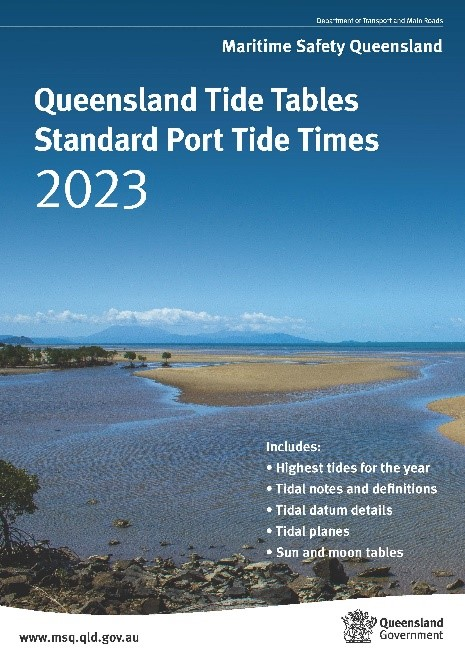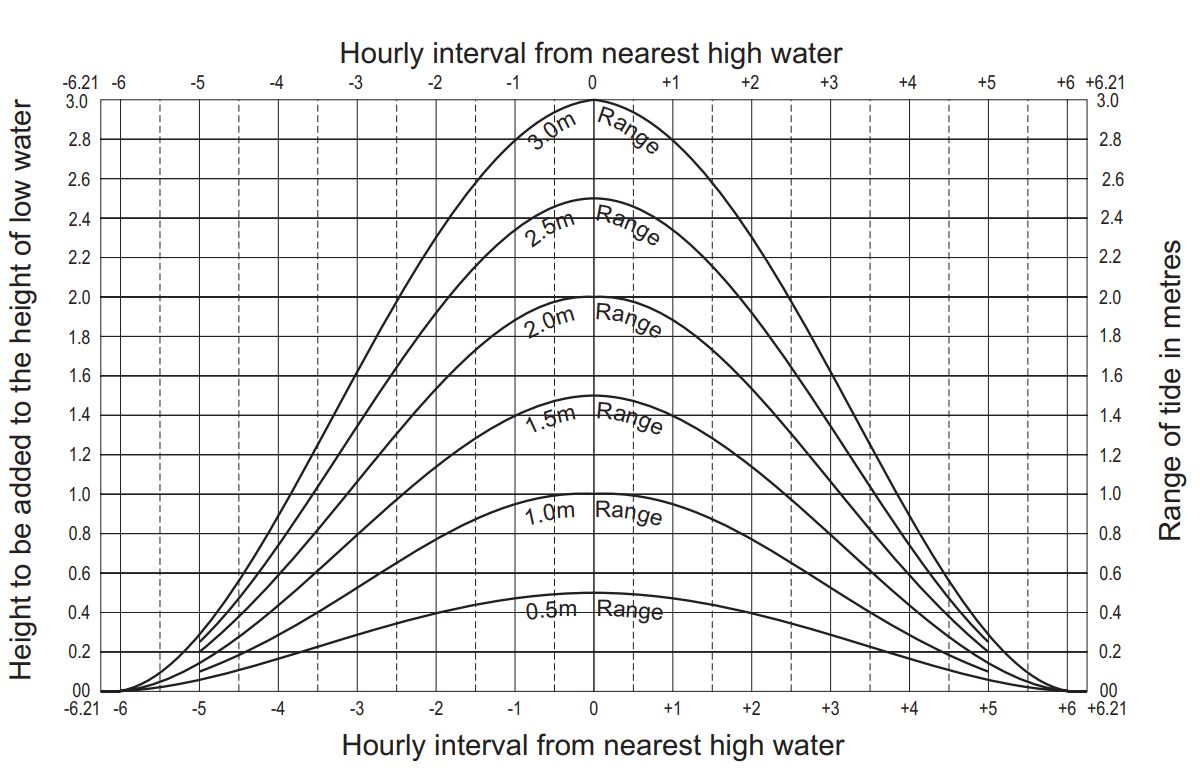# Calculating secondary port tide timesSecondary places are locations with similar tidal patterns to a standard port for which tide times and heights can be estimated by applying ratios and offsets to the tides at the adjacent standard port, provided some tidal analysis has been performed at the secondary place.

Find the required locality in the table semidiurnal tidal planes or the table diurnal tidal planes and note its standard port.

Here is our approach for estimating the tide times and heights at a secondary place.

## Time of high water (high tide)

1. Note the time difference in column 1.
2. Add or subtract (as indicated by + or -) this time difference to the predicted time of high water at the standard port.

## Time of low water (low tide)

1. Note the time difference in column 2.
2. Add or subtract (as indicated by + or -) this time difference to the predicted time of low water at the standard port.

The result is the approximate time of the tide at the required locality.

## Height of high water

1. Find the height of the predicted high water at the standard port.
2. Multiply the height by the figure in column 8.
3. Add or subtract (as indicated by the + or -) the figure in column 9.

## Height of low water

1. Find the height of the predicted low water at the standard port.
2. Multiply the height by the figure in column 8.
3. Add or subtract (as indicated by the + or -) the figure in column 9.

The result is the approximate height of the tide at the required location.

## Example calculations

Note: Standard port information used throughout the following examples refers to information from the 2022 Queensland Tide Tables for Brisbane Bar. Secondary place information refers to information from the 2022 Queensland Tide Tables for Bulwer Wrecks.

### Important information for finding tide times

#### Extract from the table Semidiurnal Tidal Planes

Height above Queensland Port Datum (LAT(1992)) table

Place Latitude Longitude  Time difference  MHWS MHWN MLWN MLWS MSL Ratio Cons  HAT
South East HW LW
1  2  3 4 5 6 7 8 9 10
HM  HM  m m m m m   m m
Standard 27 05 152 07  Standard  Port  2.22 1.84 0.81 0.42 1.32 1.00 0.00 2.78
Secondary 27 05 153 22 -0 25 -0 30 1.80 1.49 0.65 0.34 1.07 0.81 0.00 2.26

Tide times for March 16 2022 at the standard port

Time Metres
02:09 0.56
08:29 2.39
15:04 0.60
20:44 2.00

Secondary place differences to standard port

Time Metres
Time difference high water -0 25
Time difference high water -0 30
Column 8 0.81
Column 9 +0.00

### Example calculation to find time and height of high and low water at a secondary port

#### Predicted high water at secondary place calculations

 Predicted high water at standard port 2.39 m at 08:29 Time of high water at secondary place = 08:29 – 25 minutes = 8:04 Height of high water at secondary place = (2.39 x 0.81) + 0.00 =1.94 + 0.00 = 1.94 m

The first high water at the secondary place on 16 March 2022 is predicted to occur at 08:04 and reach a height of 1.94m above Queensland Port Datum (LAT(1992)).

#### Predicted low water at secondary place calculations

 Predicted low water at standard port 0.56 m at 02:09 Time of low water at secondary place = 02:09 – 30 minutes = 01:39 Height of low water at secondary place = (0.56 x 0.81) + 0.00 = 0.45 + 0.00 = 0.45 m

The first low water at the secondary place on 16 March 2022 is predicted to occur at 01:39 and reach a height of 0.45m above Queensland Port Datum (LAT(1992)).

### Calculating tide height between high and low water

Find the height of high and low tide at the Standard port and secondary place at 6:40am on March 16 2022.

#### Standard port calculation

Step 1: Obtain the tidal predications from the tables at the Standard Port.

Refer to tide times for March 16 2022 at the standard port within the important information section.

Step 2: Calculate the height difference.

Measurement
Metres
High water 2.39
Low water -  0.56
Range (height difference) 1.83

Step 3: Find the tidal curves for your range.

If the required time is 06:40 hours or 6:40 am (1 hour and 49 minutes before high water), we will use the range from step 2 to calculate the movement of a tide over a complete tidal cycle.

Using the 1.83m range to 1 hour 49 minutes before high water, you read the height at this point as 1.6m.

Standard tidal curves — tidal ranges up to 3mStep 4: Add the height obtained in step 3 to the height of low water at the standard port.

Measurement
Metres
Low water 0.56
Tidal curves  +  1.60
Range (height difference) 2.16

At 6:40 am Wednesday 16 March 2022 the tide at the standard port was approximately 2.16m.

#### Secondary place calculation

Step 1: Calculate the high and low water times and the heights for the secondary place.

Time Metres
01:39 0.45
08:04 1.94

Step 2: Calculate the height difference.

Measurement
Metres
High water 1.94
Low water -  0.45
Range (height difference) 1.49

Step 3: Find the tidal curves for your range.

If the required time is 06:40 hours or 6:40 am (1 hour and 24 minutes before high water), we will use the range from step 2 to calculate the movement of the tide over a complete tidal cycle.

Using the 1.49m range to 1 hour 24 minutes before high water, you read the height at this point as 1.3m.

Standard tidal curves — tidal ranges up to 3mStep 4: Add the height obtained in step 3 to the height of low water at the secondary place

Measurement
Metres
Low water 0.45
Tidal curves  +  1.30
Range (height difference) 1.75

At 6:40 am Wednesday 16 March 2022 the tide at the secondary place was approximately 1.75m.